Miscellaneous

Chapter 14 Class 11 Probability
Serial order wise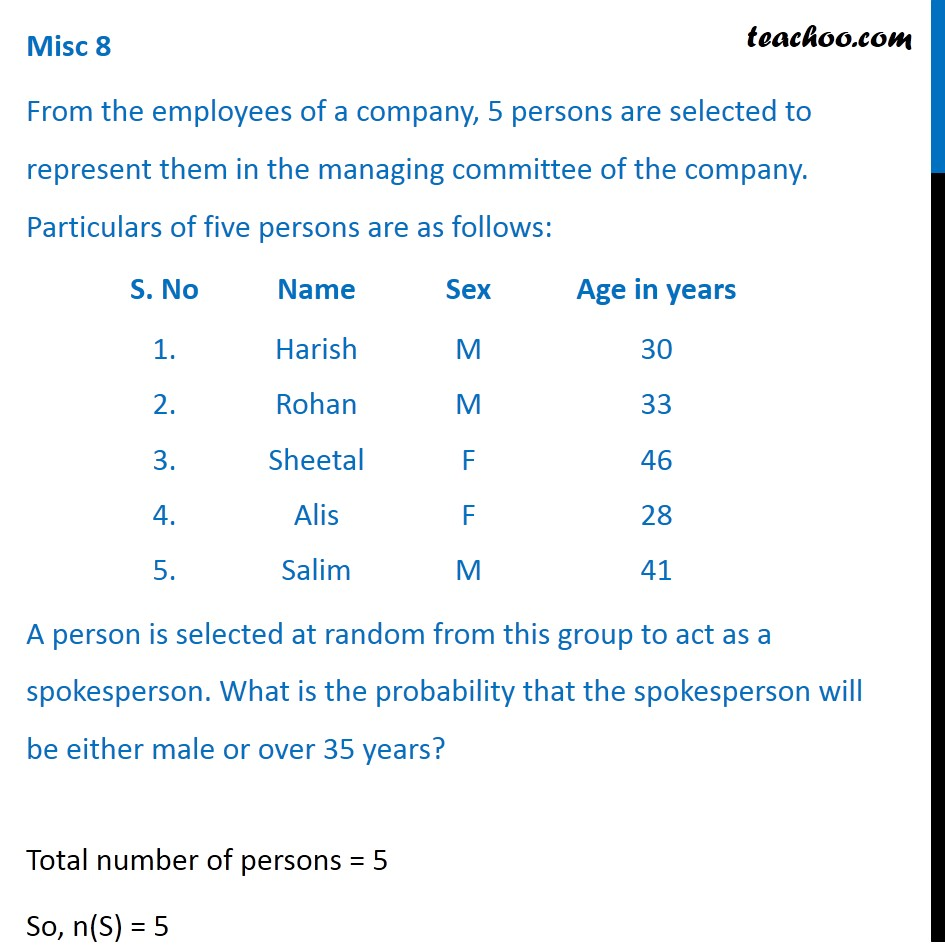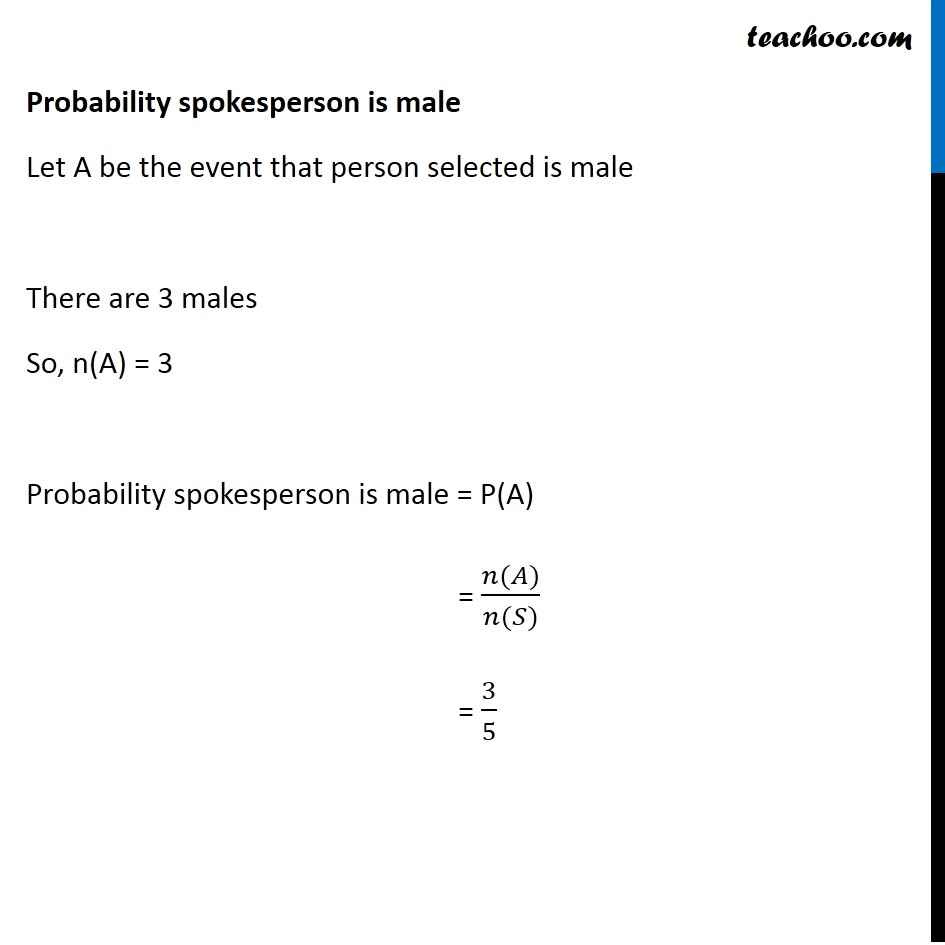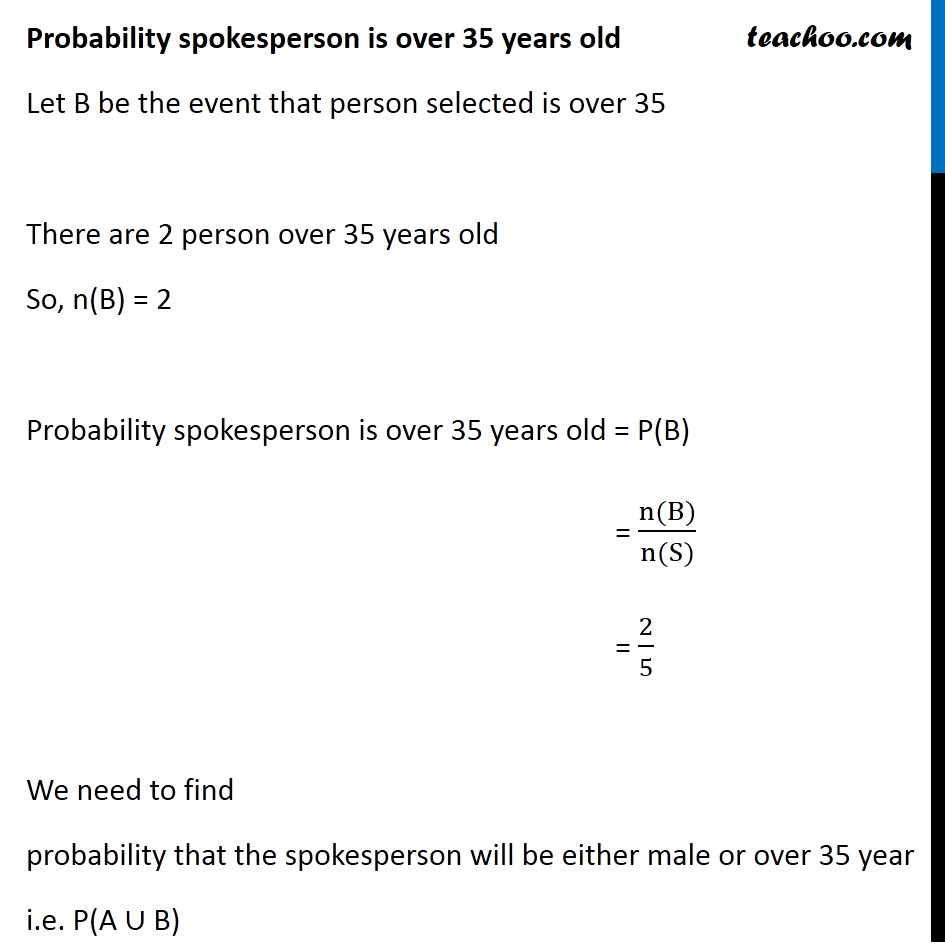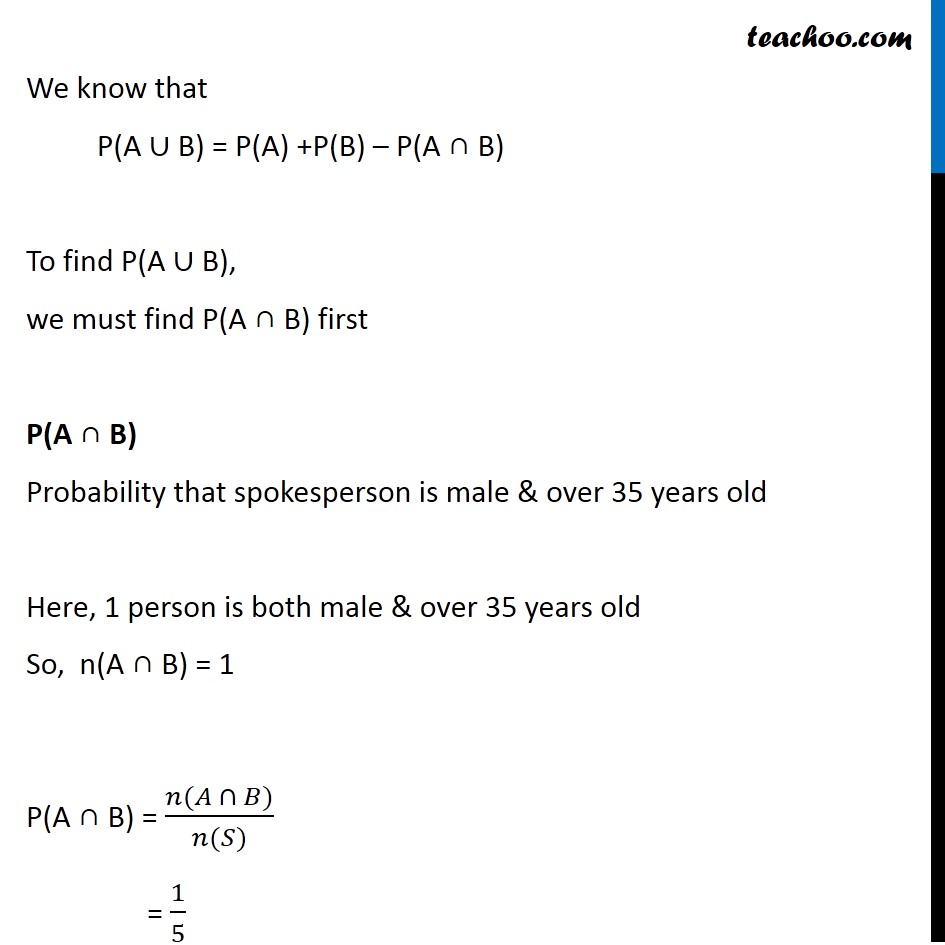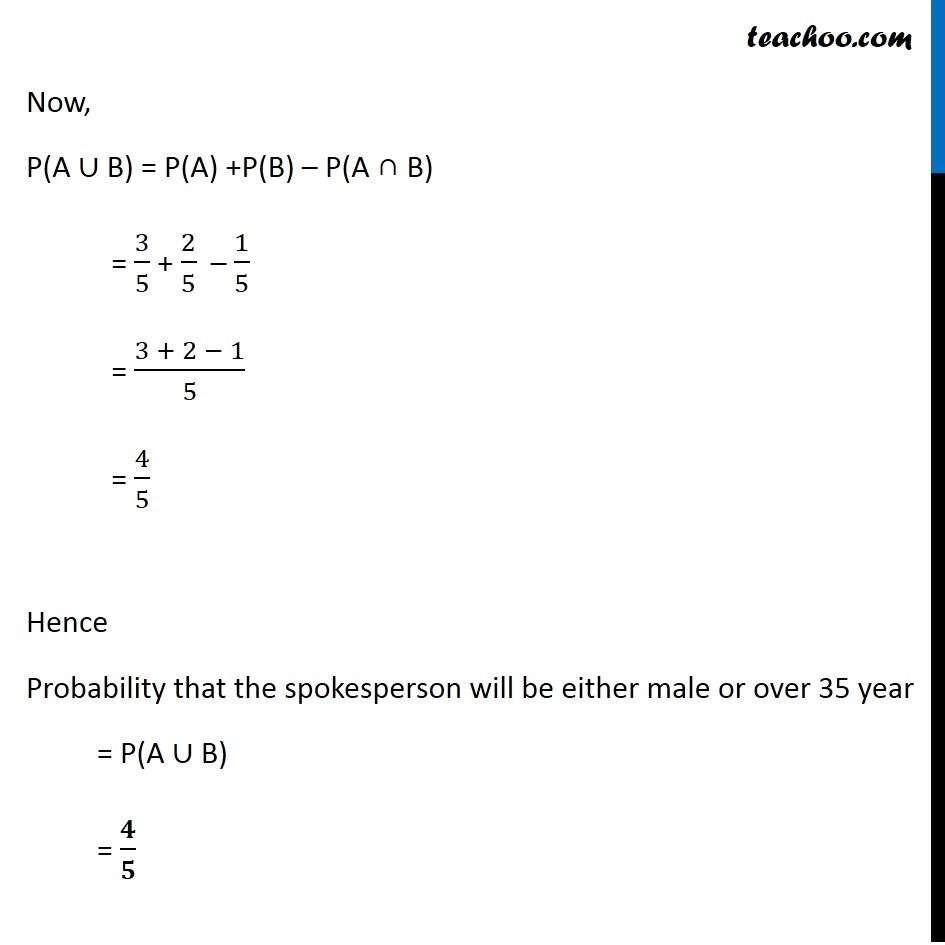Learn in your speed, with individual attention - Teachoo Maths 1-on-1 Class

### Transcript

Misc 8 From the employees of a company, 5 persons are selected to represent them in the managing committee of the company. Particulars of five persons are as follows: A person is selected at random from this group to act as a spokesperson. What is the probability that the spokesperson will be either male or over 35 years? Total number of persons = 5 So, n(S) = 5 Probability spokesperson is male Let A be the event that person selected is male There are 3 males So, n(A) = 3 Probability spokesperson is male = P(A) = (𝑛(𝐴))/(𝑛(𝑆)) = 3/5 Probability spokesperson is over 35 years old Let B be the event that person selected is over 35 There are 2 person over 35 years old So, n(B) = 2 Probability spokesperson is over 35 years old = P(B) = (n(B))/(n(S)) = 2/5 We need to find probability that the spokesperson will be either male or over 35 year i.e. P(A ∪ B) We know that P(A ∪ B) = P(A) +P(B) – P(A ∩ B) To find P(A ∪ B), we must find P(A ∩ B) first P(A ∩ B) Probability that spokesperson is male & over 35 years old Here, 1 person is both male & over 35 years old So, n(A ∩ B) = 1 P(A ∩ B) = (𝑛(𝐴 ∩ 𝐵))/(𝑛(𝑆)) = 1/5 Now, P(A ∪ B) = P(A) +P(B) – P(A ∩ B) = 3/5 + 2/5 – 1/5 = (3 + 2 − 1)/5 = 4/5 Hence Probability that the spokesperson will be either male or over 35 year = P(A ∪ B) = 𝟒/𝟓